6. Introduction to Nonlinear Programming

6.1 Nonlinearities in the model

In Section 1, we discussed linear programming models. Linear programming has many applications. Linear programming models are used in business, industry, production, agriculture, administrative and other branches of human economic and social activities.

However, not all decision models can be formulated by means of linear programming models. One reason for this is that decision environments can have uncertain outcomes. If the uncertainty in a decision environment is important, the model must incorporate that uncertainty into the analysis. Uncertainty can be described by means of a nonlinear function. Thus in nonlinear model at least one of the restricting conditions and/or objective function is nonlinear.

An important example is the management of investment portfolios in finance, where the objective might be to minimise the risk for a given expected return. Risk is measured as the variance of the portfolio, and the variance is a square (nonlinear) measure. In marketing models, the effect on sales of different amounts spent on advertising is generally a nonlinear function. Hence, linear programming cannot be used for these problems.

Fortunately, there are generalised optimisation techniques that can be used to solve many of these types of problems. One approach is to use mathematical analysis or calculus. Using calculus, one can optimise a function by taking the derivative, setting it equal to zero, and then solving. If there are constraints, a technique called Lagrangian multipliers is used. This approach is limited to relatively small problems with rather simple functions. There are also a wide variety of procedures developed for specific nonlinear models. For example, quadratic programming is a technique for solving problems with linear constraints but a quadratic objective function.

Another reason is that some constrained optimisation models violate the assumptions of a linear programming model. Two such frequent violations are:

• introducing a nonlinear function into restricting conditions or/and objective function
• restricting the values of some or all variables to integer values
In this section, we will discuss the solution of constrained optimization models that obtain nonlinear functions among restricting conditions or which have nonlinear objective function.

6.1.1 An illustrative example

An illustrative example can be the "Case of a chemical company" .

In this model, for example, the relationship of the yield and the production rate to operating conditions is not linear. For example, let us consider the number of employees who begin at each starting time: you can not have 3.7 people start at midnight. All of the variables are continuous for a linear programming model, but a fractional variable value doesn't make sense in personnel scheduling.

Simplex process guarantees no integer values for the solution vector. There is no a priori reason why any vertex of the polyhedron of feasible solutions should be a lattice point, i.e., have integer coordinates. Approximating the coordlinate values with integers does not necessarily yield a feasible vector, since the vertex yielding a solution may be nearest to a lattice point outside the region of feasible solutions; and the nearest feasible lattice point may not be near enough to justify any approximation.

6.1.2 Integer programming model

For many decision problems, some of the variables must be restricted to integer values. Constrained optimization models can violate either the linearity assumption or the continuous variable assumption.

6.1.3. How to solve nonlinear programm

Nonlinear programming model can not be solved by linear programming software. The software of linear programming assumes that all of the functions are linear and will not accept any other input. The simplex algorithm does not solve nonlinear programming models. For the solution of nonlinear programmes special algorithms and software has to be used.

An integer linear programming model is a linear programming model in which some of the decision variables are restricted to integer values. Such model can be solved by means of simplex method. We can solve an integer nonlinear programming model by the simplex method and hope that the variables will have integer values in the optimal solution. In some cases we can be lucky but most of the time this does not happen.

In general, software based on nonlinear programming algorithms is less widely available than software for linear programming models and integer programming models.

6.1.4 Classification of nonlinear models

Nonlinear programmes can be classified according to

a) presence of nonlinearity among restricting conditions

b) algorithms (software) used for solution

 Restricting conditions Objective function Algorithms Methods General nonlinear programming model nonlinear nonlinear gradient methods (Excel Solver) Quadratic programming linear nonlinear - quadratic form Zoutendijk Frank-Wolfe Wolfe Integer programming integer values in results complete enumeration cutting-plane branch and bound Piecewise programming nonlinear functions are reformulated to piecewise functions simplex method

Most used software is Solver (Excel). This software gets approximate solutions which are good enough for the practice.

6.2 General nonlinear programming models

6.2.1 Definition of the problem

The nonlinear programming problem is concerned with the maximisation or minimisation of a continuous and differentiable function of a real variables

f(x1, x2, …, xn)

called objective function, subject to m inequality constraints

gi(x1, x2, …, xn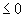, i = 1, 2, …, m, and xj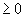, j = 1, 2, …, n.

Both nonlinear restricting conditions and objective function can be depicted as nonlinear lines, see Fig. 1: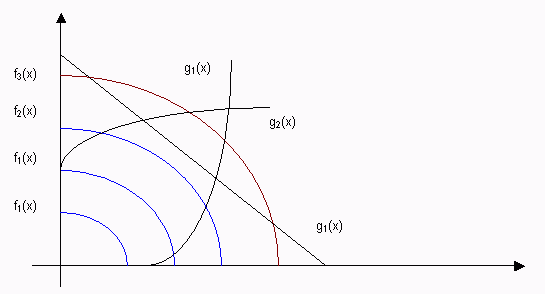Figure 1

The functions gi(x1, x2, …, xn, i = 1, 2, …, m, will be assumed to be convex. The last corollary asserts that the feasible region is convex. In general, solving general problem requires that a point from the feasible region can be found which es f. The objective function f will be assumed to be convex (concave) if problem involves the minimisation (maximisation) of the objective function f. These restrictions on f and gi together lead to an important property of the problem:

Any local optimum is a global optimum of the objective function in the feasible region.

This follows from the fact that the line segment joining a local optimum attained in the region to a global optimum also attained in the region must itself lie in the region because of convexity. Hence f must be a constant on this segment Thus a local optimum is global. Illustration of the property is seen on Figure 2: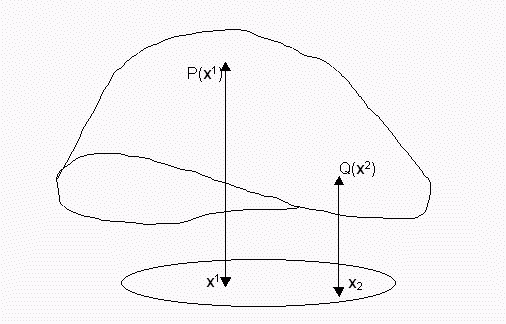Figure 2

The nonlinear function (depicted in Figure 3) reaches its maximum on inner region of its set of feasible solutions at the point x1; this maximum is equal to P(x1).The minimum is reached at the boundary point x2 with the value of Q(x2).

Ordinary differential calculus methods for finding the extremum of an unconstrained function, when applied to f, may yield values which are in the region, in which case, one has a solution of the problem. One usually begins by testing for an optimum in the region. This process itself may be consuming and costly. On the other hand if the optimum lies on the boundary, then the problem is no longer simple. If it were known which of the constraints determined the optimum, then one could apply the Lagrange-multiplier method using these "active" constraints after replacing the inequality sign by equality, since the optimum is on the boundary. In general, exploration of the boundary is necessary for determining the active set; this may be tedious when m is large. The purpose of all efficient methods is to use steps which lead to active constraints and, in fact, to the optimum.

6.2.2 Examples

Examples of nonlinear programming problems abound in the literature. The following are among the better-known problems:

A monopolist wishes to maximise his revenue. The sale price pj of a commodity depends on the quantity of each of several commodities which he produces unlike the case of free competition. Thus, if the revenue is given by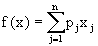where pj is the price of the j-th commodity , and xj is the amount of the j-th commodity to be produced, then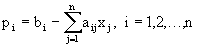If we substitute these quantities in f, we obtain a quadratic function to be maximised subject to nonnegativity constraints x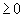, j = 1, 2, …, a and subject to capacity constraints which may be prescribed linearly. Thus, since f (x) is nonlinear, we have a nonlinear program.

A similar problem may be formulated in which the cost of producing a commodity depends on the quantity produced. In this case the objective is to find the xj which minimise the quadratic function f(x) subject to xand subject to the constraints that the entire capacity should be used. These constraints can be expressed in terms of linear inequalities with nonnegative coefficients.

Another interesting example is to find a best linear approximation to a set of observed data (xj, yj), j = 1, 2, …, n, subject to constraints on the coefficients of the approximating linear expression. Thus the problem may take the following form:

Find the a and b which minimise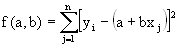subject to a, b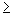0. Ordinary least-square methods may not be applicable because of the presence of constraints.

Yet another example is that of portfolio selection, in which an investor wishes to allocate a given sum of money C to different securities. He must maximise the expected return for a fixed variance or minimise the variance for a fixed return. If xj, j = 1, 2, …, n is the amount to be invested on the j-th security, then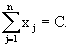The total return is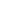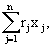its expected value is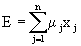and its variance is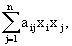where (aij) is the covariance matrix of random variable rj, the return on the j-th security, and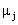is its expected value.

These problems are obviously nonlinear, in the first case because of the presence of a quadratic constraint, and in the second because this constraint is the function to be minimised.

Problems in which profit is described by a nonlinear function of the variables to be maximised subject to constraints can also be nonlinear programs.

6.2.3 Background to the solution

Necessary and sufficient conditions for an optimum on the boundary are described in general Theorem for nonlinear programme - Kuhn-Tucker theorem. These conditions occasionally enable one to -obtain this optimum. However, they provide basic ideas used in developing iterative algorithms. This characterisation is obtained through concepts involving gradients and saddle points and through differential equations. The greater the variety of characterisation theorems, the greater is the possibility of constructing ingenious algorithms, perhaps by borrowing ideas from related fields.

We shall be using the Lagrangian function F(x,u) for a maximisation problem. This function is defined by following formula:

F(x,u) = f (x) - uG(x)

where u = (u1, …, um) is vector of lagrangian multipliers, f(x) is the objective function and G(x) is the system of restricting conditions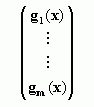Here x = (x1, …, xn) and G(x0 implies that all gi(x0.

Now let´s define gradient of G(x)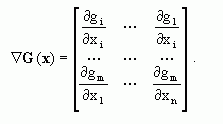.
Note that if x is a point on the boundary, then it need not yield equality for all the constraints. Since it is a feasible point, the constraints on whose boundary it does not lie are satisfied strictly; that is, gi(x) < 0. The remaining constraints gi(x) = 0.

6.2.4 Kuhn-Tucker theorem

Kuhn-Tucker theorem implies, that

A necessary condition that f(x) attains its maximum at a boundary point xo of G(x) < 0, with x > 0, is that there exist u0 such that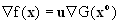The theorem obviously also holds if x is an interior point, for in this case x > 0, G(x) < 0, so that u = 0.

The point (xo,u) is called a saddle point of F(x,u).

6.2.5 Kuhn-Tucker conditions

A necessary condition for (x,u) to be a saddle point of F is that x and u satisfy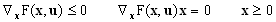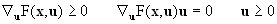The foregoing two sets of conditions, together with the two conditions analogous to series expansions in the neighborhood of (x, u) up to linear terms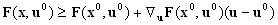are sufficient for (x,u) to be a saddle point with x0, u0.

Kuhn-Tucker conditions reduce the problem of maximising a function subject to constraints to that of minimising a single function, i.e., the lagrangian, with respect to u after taking its maximum with respect to x. Thus one is able to apply iterative techniques to minimise the lagrangian with respect to u.

6.2.6 Kuhn-Tucker theory in process of solution

Kuhn-Tucker conditions are the base for many solving procedures. An iterative procedure that converges to the optimum may be employed in solving the nonlinear problem. We select an arbitrary point in the feasible region as an initial point; if it does not yield the optimum, we obviously would like to shift to another point at which the objective function has an improved value. Often the gradient determines the direction of our movement. In a maximisation problem we move along the gradient; otherwise we move in a direction opposite to that of the gradient. The actual distance to be traversed may be determined in a manner analogous to that used in the method of steepest descent. By repetition of this process, the optimum may be reached in a finite or an infinite number of steps. Several of the algorithms discussed were actually employ variations of the procedure just described. We illustrate with examples.

For convenience we consider the problem of minimising a convex function. Hence at each step we calculate -f instead off. The initial step towards the solution involves a routine check by differentiation to determine whether the maximum lies in the interior of the feasible region R. If not, we know it lies on the boundary.

We start with any point in the feasible region and calculate -f(). If is an interior point, we move along the vector -f(xo) a distance equal to its length and arrive at a point whose coordinates are

x1 = xo -f(xo).

If x1 belongs to the region (i.e., satisfies the constraints) and f(x1) < f (x°), then x1 is our new point. Otherwise we obtain a new point by applying the gradient method on the segment (x°, x1). If, in either ctase, x1 does not belong to the region, we take those constraints which are not satisfied by x1 and calculate their intersection with the line passing through x° and x1. This line in n space is given as the intersection of n-1 hyperplanes. Hence for each constraint one must solve for n unknowns from n. equations, of which n-1 are linear and the remaining one is a constraint.

Among all these points of intersection, the one nearest to x° must satisfy all the constraints and, hence, would belong to R. We denote this point by x1. If f (x1) < f(xo), then x1 is the new starting point; otherwise we apply the gradient criterion to pick a point on xo, x1 at which the value of f would be smaller than at xo.

If xo lies on the boundary of R and is the required optimum, the gradients of the objective function and the constraints (evaluated at xo) satisfy Kuhn-Tucker theorem. Otherwise we compute the projection of -f on the intersection of the tangent planes to all the surfaces gi(x) = 0 at xo. The equation of such a tangent plane is given by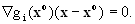The intersection of these planes is a plane of dimension at least equal to n-m. The projection of -f on this intersection is a line whose length is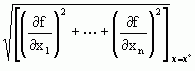This projection determines a ray along which we must move to our next point. The magnitude of the step is determined by the gradient criterion. If this new point does not lie in R [if the g:(x) are convex, the point cannot lie in R], then a new appropriate point may be chosen in R such that f shows an improvement for the new value. This procedure of choosing the point in R is involved and is discussed by Rosen.

6.2.7 Example

In the example considered below, we shall only show how to obtain the length of the projection of -f on the tangent planes. If the projection of -f on the intersection of these hyperplanes is zero, we locate the surfaces which, if dropped from the set of gi(x) containing x°, will yield a nonzero projection of the gradient on the intersection of the tangent planes to the remaining surfaces. Once this is accomplished, the procedura is the same as above.

To illustrate, let

f(x,y) = (x - 3)2 + (y - 1/2)2
g1(x,y) = x - 2
g2(x,y) = x2 - 2x - 3 + 4y

and suppose it is desired to minimise f subject to gi0, i = 1, 2.

Since f is convex, a local minimum is a global one.

Let xo = (1,1); then -f (x°) = (4, -1). The farthest we can go along the direction determined by -f(x°) is x1 = (2,3/4), which is on the boundary of both constraints; i.e., the line pierces the intersection of both constraints at this point. The reader should show that the projection of (2, -1/2) on x - 2 = 0 is (0; -1/2). This furnishes the direction. Using the gradient method, we substitute in f the quantity x1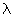multiplied by the projection off(x1), that is, we put

x = 2+.0 y = 3/4 -/2

and minimise with respect tofor 01. We obtain= 1/2. Thus our next point is (2,1/2) (obtained by putting= 1/2 in x and y), which gives the required minimum by Kuhn-Tucker theorem. The minimum value of f is 1.

6.2.8 Best known algorithms

Following list presents a collection of algorithms for solving nonlinear programmes bearing the names of their respective inventors.

• Frank and Wolfe´s method.
• Wolfe methods (the simplex method of quadratic programming).
• Carroll´s Technique with Development by Fiacco and McCormick.
• Zoutendijk´s Method of Feasible Directions.
In practice is used "Solver" in MS-Office packet which yields good enough approximate solutions.

6.2.9 Piecewise programming models

The nonlinear programming models can be reformulated to those with piecewise linear objective functions. These functions are nonlinear. However, they are linear over "pieces."

A piecewise linear function consists of straight line segments that are connected at the end points. The breakpoints are the variable values where the slope of the line segments changes. The slope of the objective function is called the objective function rate.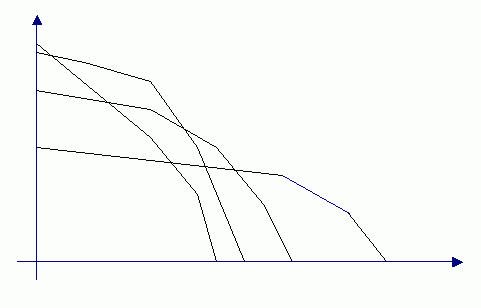Figure 3

On Figure 3 are nonlinear curves replaced by piecewise linear lines. It enables use some modification of simplex method to obtain solution.

Frank and Wolfe consider the problem of maximising a concave quadratic function f = ax - xAx subject to linear constraints Bxb and x0, where A = (aij) is an n x n positive-semidefinite symmetric matrix, B = (bi;) is an m x n matrix, b = (b1, …, bm), x = (x1, …, xn) and a = (aij).

The algorithm is based on the Kuhn-Tucker Theorem and uses the simplex method of linear programming. Geometrically, the Kuhn-Tucker Theorem implies that f has a local extremum at x if and only if the normal hyperplane to the gradient vectorf at x is a locally supporting hyperplane of the constraint set. A hyperplane touching the constraint set at a point x satisfies the above condition if and only if x has the maximum projection along the outward normal to the hyperplane. Since f has been taken to be a quadratic function, the expression for an extremum on the boundary can be written as a linear function of x, and the simplex method can be used to arrive at the optimum.

Wolfe furnishes a variation of the above technique.

Step 1: Begin with the set of relations

Bx + w = b
Ax - v + Bu + z1 - z2 = -px
{v, u, z1, z20

where z1, z2are two successive iterations, and w is vector of penalty variables.

Step 2: Use the simplex method to minimise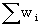to zero, keeping v and u nonnegative. Discard w and the unused components of z1 and z2. Let the remaining n components be denoted by z, and their coeffcients by E. A solution of the system is

Ax = b
Ax - v + Bu + Ez = - up
{x, v, z0

Step 3: Simplex method is used to minimise objective function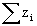subject to folllowing side conditions:

For k = 1, . . . , m, if xk is in the basis, we do not admit vk; if vk is in the basis, we do not admit xk. It will take at most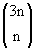iterations to reach the solution. The x component of the terminal basis solution is a solution of the quadratic problem.

Wolfe´s method is available in software LINDO, QSB. Also Solver (part of MS Excel(R)) can be used to obtain solution with preassigned accuracy.

6.3.1 Example

6.4 Integer programming models

6.4.1 Introduction to integer programming

The category of mathematical programming includes a wide variety of techniques used to solve optimisation problems. The decision maker is trying to maximise or minimise some objective, subject to a set of constraints. The most widely used technique in this category, of course, is simple linear programming. This has been discussed in most applications

Integer programming allows some or all of the decision variables to be integer valued while keeping linear relationships in the objective function and constraints. The solution integer programmes can be successful using the Excel-Solver package.

6.4.2 Example

Many business problems could be suitably formulated and solved by linear programming, except for one drawback: they require solutions that are integers. For example, a variable xj in a linear programming model may refer to whether or not a new plant is built. If xj = 1, the plant is to be built; xj = 0 means no plant; and a value of xj anywhere in between (xj = 0,3 for instance) makes no sense. There is no guarantee that the standard solution procedures for linear programming will give an integer solution.

6.4.3 All and mixed-integer programming

To deal with integer programming models, a set of techniques has been developed.

1. Some problems may require that all variables in the problem be integers. In this case we call the problem the all-integer problem. A special case of the all-integer solution occurs when all the variables can take on values of only 0 or 1.

2. The most general case of integer programming is that in which some variables are required to be integers, but others can take on any values. This is called the mixed-integer programming problem.
An important exceptions to this are the solutions of network problems by LP, transportation problem, assignment problem which do give integer solutions.

6.4.4 Principles of formulating integer programmes

In general, integer programming problems are formulated in much the same way as the standard linear programming problems discussed in earlier sections, with the added proviso that one or more variables must assume integer values. That is, we establish a linear objective function that is maximised or minimised subject to linear constraints. However, there are some uses of zero/one variables that are unique to integer programming. A zero/one or binary variable is one that can take on only values of 0 or 1. The value 1 can be used to indicate the presence of something (a factory, for example); 0 indicates its absence. The use of binary variables is illustrated in example.

6.4.5 Example of the Fixed-Charge Problem

Suppose a given product, if it is produced, contributes \$50 per unit to profit. How-ever, a one-time expense of \$1000 is incurred in setting up the machinery for a production run. This cost is zero if no units are produced.

Let x be the number of units produced. Then we could formulate the problem so that: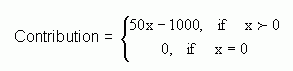However, Contribution is not a linear function of X in this formulation.

To rewrite the formulation in a linear form, we introduce a new variable y, which takes on only the integer values of 0 or 1. Then we maximise (50x - 1000y) subject to whatever other constraints we have plus the following two:

y < 1 and y is an integerMy

where M is penalty cost (some very large number).

Note that when y = 0, the second constraint forces x to be 0 and the contribution (50x - 1000 is 0 also; when y = 1, there is no practical limit on x; moreover, the fixed-charge amount \$1000 is deducted from the profit. Hence, the use of the zero/one or binary integer variable y allows us to formulate this type of problem with linear constraints.

The fixed-charge type of problem is common in business. There is an investment cost to build a new plant before any production can occur. There are usually fixed costs that have to be incurred if a second shift is undertaken or a new warehouse is opened. The problem is basically linear but also similar nonlinear model of the Fixed-Charge problem can be formulated.

6.4.6 Formulation of integer conditions in spreadsheet

Another approach to formulating this fixed-charge cost might be to use a spread-sheet "IF" function. Suppose, for example, the number of units produced (the x value) were in cell D2 on the spreadsheet. Then the total cost might be written as:

=IF(D2>0, 50*D2-1000, 0).

While this is a logically correct formulation, it also is not a linear function, and would not be appropriate for a linear model.

6.4.7 Solution of Integer programming Problems

Ordinary linear programming problems are solved by the simplex method, which very efficiently produces optimal solutions even for large problems. However, these solutions are not necessarily integer. Sometimes, of course, a noninteger LP solution can be rounded off appropriately to give an integer solution. If the rounded solution is feasible and has a profit close to that obtained for the noninteger problem, the rounding procedure is likely to be adequate. However, for many important problems, this procedure will not work. In particular, if the problem contains zero/one variables of the type illustrated in the formulations earlier in this section, rounding will generally give infeasible solutions. Hence, a solution procedure designed for integer problems is required.

When a problem is required to have an integer solution, it means that there are a finite or counting many numbers of possible solution points.

1. One approach is to use complete enumeration method and evaluate every possible solution to find the optimum. If there are only a few integer variables, enumeration may be feasible and an efficient procedure. However, for most realistic problems, the number of possible solutions is very large and complete enumeration is not computationally realistic.

2. Branch and bound methods. Complete enumeration might not be necessary if we could find ways of eliminating whole groups of solutions from consideration. The branch and bound technique is a method for doing this. Basically, the potential solutions are organised in a tree or hierarchy. A method is used to find a bound for a subset of these solutions. A bound is an upper limit (in the case of profit maximising) or lower limit (for cost minimisation). If a feasible solution can be found that is better than the bound for the subset, the entire subset can be eliminated. The process continues until the optimum is found, the solution that is better than the bounds on all the subsets.

3. Another technique is called the cutting-plane method. It is a variant of the simplex method, and in fact starts with the simplex solution to the linear programming problem, ignoring the integer requirements. Then new constraints (cutting planes, or simply cuts) are added to the problem that make infeasible the previous noninteger optimal solution but do not exclude any feasible integer solutions. A new LP solution is obtained and the process repeated (i.e., new cuts added) until an integer solution is found. This method is less widely used than the branch and bound method.

6.4.8 Using Solver for Integer Programming Problems

Solver is a package incorporated in the Excel and Quattro spreadsheets. Its use in solving ordinary linear programming problems has been illustrated in earlier sections. Solver also has the ability to solve integer programming problems. One simply has to identify which variables are to be integer valued. The use of Solver is illustrated for the factory size and location problem formulated earlier.

After start up the Solver a Solver Parameters Dialogue Box appears:

1) Target Cell (Objective)
2) Objective: minimise/maximise
3) Changing cells (decision variables)
4) ConstraintsConstraintsConstraints =
Check linear model in options dialogue box !

1) Denote cell in which is expected optimum located in the spreadsheet (e.g. value "z").

2) Type minimise or maximise

3) Denote cells (columns) in which coefficients at decision variables are located.

4) Denote cells (rows, columns) in which corresponding constraints are located.

The Use of Solver is illustrated by example Optimal Factory Size and Allocation.

6.4.9 Problems with Solver

Because it uses the branch and bound procedure that involves solving a sequence of linear programming problems, using Solver for integer problems takes much longer than it does for ordinary LP problems. Also, the Solver Reports are not of value as they are in ordinary LP. There is no Sensitivity Report, and the Answer Report merely gives the same information as displayed on the spreadsheet.

While nonlinear programming methods are powerful solution procedures, the user should be aware of some limitations. The most important is that the procedure may produce a local optimum rather than the global optimum. There is no guarantee in general nonlinear programming that the highest peak (global optimum) will be found. This is an important concern. One approach is to solve the problem several times, each time starting from a very different initial solution. This will increase the chance that the global optimum will be found.

A second problem that is sometimes encountered is that no solution may be found. This can occur because of the way the search process works, the process may take a step that goes over a cliff and cannot recover). In spreadsheet models such as Solver, a trial solution may cause trouble with some of the functions or equations in the model-trying to divide by zero or trying to take the logarithm of a negative number. Such problems will cause the solution process to stop without reaching an optimum. Finally, scaling may cause a problem in model solution.

All computer codes compute with a certain degree of accuracy. If a model has coefficients that vary greatly in magnitude (e.g., one number being 0,00000023 and another 123,345,210), computational difficulties may occur. This can be handled by rescaling the values: convert to millions or billions, for example, for one or several variables.

6.4.10 Summary

Models that contain nonlinear objectives or constraints (or both) may be solved by general optimisation methods. These usually involve a search process generating a sequence of trial solutions. Some newer methods create successive "generations" of trial solutions. Computer programs are widely available for solving these problems, including ones for personal computers. Some caution in the use of general optimisation methods is necessary.